﻿ Multistability in a Single System with Hidden Attractors- Theory and Experiment

Multistability in a Single System with Hidden Attractors- Theory and Experiment

Papri Saha, Dolonchampa Saha Anirban Ray, A. Roy ChowdhuryOPEN ACCESSPEER-REVIEWED

Multistability in a Single System with Hidden Attractors- Theory and Experiment

Papri Saha1, Dolonchampa Saha Anirban Ray2, A. Roy Chowdhury2,1Department of Physics, B.P. Poddar Institute of Management & Technology, VIP Road, Kolkata, India

2High Energy Physics Division, Department of Physics, Jadavpur University, Kolkata, India

Abstract

Existence of more than one attractor for a single nonlinear system and the corresponding generation of multistability is investigated both from the point of view of theory and experiment. The system under consideration is relatively a new one which possess a single stable fixed point but shows many characteristic features of attractors in phase space. It is shown that the change in the initial condition triggers a host of phenomena not observed before in any dynamical system. Change in the initial conditions enables a switch over from one attractor to the other. Our analysis clearly shows the changes in the Poincaré section and the mechanism of formation of unstable periodic orbits. We point out that in spite of these peculiarities, the model does not possess any standard route to bifurcation but one can visualize the change in the periodicity with respect to the parameters and its dependence on the initial conditions. In the next half of our paper we have constructed analogue electric circuit for the equation and have introduced a mechanism for the choice of initial condition with the help of relay in the circuit. These modified circuits were then used to simulate experimentally the sensitivity on the initial conditions and the transition from one to the other attractor.

At a glance: Figures

12345
Prev Next

• Saha, Papri, Dolonchampa Saha Anirban Ray, and A. Roy Chowdhury. "Multistability in a Single System with Hidden Attractors- Theory and Experiment." International Journal of Physics 2.6 (2014): 217-225.
• Saha, P. , Ray, D. S. A. , & Chowdhury, A. R. (2014). Multistability in a Single System with Hidden Attractors- Theory and Experiment. International Journal of Physics, 2(6), 217-225.
• Saha, Papri, Dolonchampa Saha Anirban Ray, and A. Roy Chowdhury. "Multistability in a Single System with Hidden Attractors- Theory and Experiment." International Journal of Physics 2, no. 6 (2014): 217-225.

 Import into BibTeX Import into EndNote Import into RefMan Import into RefWorks

1. Introduction

In recent years a new phenomenon called multistability has attracted the attention of many researchers in the field of nonlinear dynamics. This phenomenon depicts the existence of more than one attractor for a system and transition from one to the other by switching the initial condition. This behaviour has been observed in various fields of science such as laser optics , condensed matter physics , reaction-diffusion systems , neuroscience , and many more. To simulate this type of situation two very similar systems are coupled just as in the process of synchronization. It is then observed that a change in the initial condition switches between the two attractors of the subsystems. Lyapaunov exponents of such a system is seen to have very strong dependence on the initial conditions. Arecchi et. al. systematically investigated multistability for the first time by performing experiments with a gas laser  and numerical simulations of the Duffing oscillator . Extreme multistability was also demonstrated in a system of two coupled identical Lorenz oscillators by Sun et al.  and recently been studied in the three-variable autocatalator model by Ngonghala et al. . It is shown in  that extreme multistability is closely related to the emergence of a conserved quantity. The conserved quantity may appear due to a particular coupling scheme that gives rise to system variables that are no longer independent. In this case, the value of the conserved quantity is determined directly by the initial conditions. Also it is described by Feudel and Grebogi that the easiest way to construct a multistable system is to take a conservative one and introduce small amounts of dissipation . Systems with time delay are also found to exhibit this process of multistability . The basins of attraction of different attractors of multistable systems are interwoven in a complex manner, and separated by one or several chaotic saddles. The dimension of the basin boundaries is very close to the dimension of the state space.

Here in this communication we report the existence of a single nonlinear system which itself has more than one attractor . It was already shown that for certain parameters, besides the point attractor and chaotic attractor, this system also has a coexisting stable limit cycle. We have considered a different scenario by varying the parameter which was kept constant earlier and observed that switching between different attractors takes place with the change in the initial condition in a wide range of this parameter. No coupling of similar nonlinear systems is required in this case. We also show that its Lyapunov exponent has strong dependence on the initial conditions. To explore the event in a greater detail we have analyzed the formation of “shrimp” like structures (these are actually periodic islands in the chaotic sea first envisaged by Gallas  and then by Lorenz ) and their change with respect to initial condition variation. This shows that the shrimp structure has notable changes. The deep reason behind all such phenomena is really a change in the mechanism of the formation of various unstable periodic orbits.

Although bifurcation has been studied in detail by various researchers but little investigation of multistability through electronic circuits can be found. Experimental observation of extreme multi-stability in an electronic system of two coupled Rössler and Lorenz system has been done by M.S. Patil et. al. But experimental demonstration of multistability through single nonlinear system has been shown yet. In the second half of the paper an electronic circuit realization of the proposed system is presented using analog electronic elements.

2. Formulation

The nonlinear system under consideration can be written as(2.1)

It may be pointed out that a similar system was studied by Sprott, where the constant ‘awas varied in a finite range and ‘bwas taken as a constant set at 4.0. An important feature of Eqn(2.1) is that it has got only one fixed point at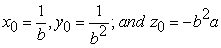The corresponding stability is governed by the eigenvalue equation(2.2)

where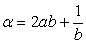The real part of the eigenvalue being negative the fixed point is stable for the entire regionand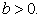Numerically, if we set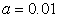and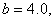these eigenvalues turn out to beOne should note thatis the case in the equation discussed in Ref . But for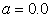the eigenvalue equation is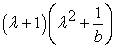so that the eigenvalues become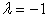and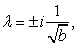if b is greater than 1 then the point is stable. This is the case of Sprott equation with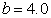One of the most interesting feature is that though there is only one fixed point yet the present equation exhibits all the features such as bifurcation, chaotic attractors of standard nonlinear systems. But the most significant difference that arises is the dependence on the initial condition, which is not usually seen in standard chaotic systems. This is actually referred to as multi-stability.

2.1. Variation of Bifurcation Diagram with Initial Conditions

Though the present model does not possess the standard routes such as pitchfork or saddle node type bifurcation yet one can sort out the variation of different periodic orbit structure with respect to the new parameter ‘b and obtain a bifurcation like structure. The different bifurcation patterns also depends in a very sensitive way on the initial conditions and it is to be pointed out that changes in initial condition may lead to different attractors. To begin with, we consider the bifurcation pattern generated when the parameter b varies. In Figure 1a, we show such an instance of bifurcation when the initial point is (x0 = 2.2, y0 = 3.1, z0 = 1.1).

Here one finds that the attractor gets destroyed just before b = 4.0 and again starts after b = 4.0. But if we change the initial condition to (x0 = 1.2, y0 = 1.0, z0 = 1.0) the corresponding diagram is given in Figure (1b) where pattern is different altogether, but with some gaps near b = 3.5 and b = 3.8.

On the other hand if we start with (x0 = 0.2, y0 = 0.1, z0 = 0.1) then the chaotic zone vanishes and we only get periodic states, though the bifurcation have started much earlier. This is shown in Figure 1c.

Next, we consider the initial values (x0 = 0.2, y0 = 0.1, z0 = 0.1) whence the chaotic states are seen again in Figure 1d. Here one observes that though the bifurcation starts near b = 2.0, yet very near to b = 4.0 we have a co-existence of the old and new attractor. Here one sees that the periodic and chaotic state can co-exist. The situation as depicted in Figure 1e remains almost the same when we consider the initial values (x0 = 0.2, y0 = 0.1, z0 = 0.1) except that minor variation in the bifurcation diagram can be observed in the range 3.75 < b < 4.5. So in all these five cases, the bifurcation parameter b is same but the change in the initial point gives their different structure and such type of events are not usually observed in standard nonlinear systems.

In Figure 2 and Figure 3, we show the variation of phase (periodic and chaotic) of the system with changing initial condition of x0 and z0. Figure 2 and Figure 3 depict that the system periodically passes through chaotic and periodic states respectively as the initial condition is changed. We kept (a = 0.01, b = 4.0) fixed for both Figure 2 and Figure 3. We took (y0 = 0.1, z0 = 0.1) and varied x. The attractor is completely destroyed when 0.1 < x < 0.5. But chaotic and periodic states alternate in rest of the region. In Figure 4, we kept (x0 = 1.0, y0 = 1.0) and vary z between 0 to 5. Here also chaotic states alternate with a purely periodic ones. Now the question arises – are those chaotic states same or different? This can be examined in a very crucial way by following their bifurcation diagram minutely for each chaotic states (defined by corresponding initial condition). We first considered x = 3.0, y = 0.1, z = 0.1 from Figure 2 and keep a = 0.01 but vary b from 1.8 to 2.4 and observed the parameter window b = 2.0 to 2.1. This is shown in Figure 4a. Then, we have considered another initial condition x = 1.5, y = 0.1, z = 0.1, a = 0.01 and look into the region near between 2.0 to 2.1 , the whole scenario changes as in Figure 4b. This is an indication to the fact that the bifurcation pattern is different in this parameter window. Furthermore, we can repeat the analysis again with both initial conditions and concentrate near b = 4.1 to 4.3. The case with x = 3.0, y = 0.1, z = 0.1 and a = 0.01 is given in Figure 4c, whereas the situation with x = 1.5, y = 0.1, z = 0.1 and a = 0.01 is reproduced in Figure 4d. The two events show wide differences and confirm that the alternate chaotic states are actually different attractors. The whole situation is beautifully depicted in Figure 4e where we show the phase space structure variation with x0, the initial value of ’x’. It shows alternating the periodic and chaotic situation with variation of initial conditions.

Figure 1. Bifurcation diagram of the system for initial values (a) x0 = 2.2, y0 = 3.1, z0 = 1.1, (b) x0 = 1.2, y0 = 1.0, z0 = 1.0, (c) x0 = 0.2, y0 = 0.1, z0 = 0.1, (d) x0 = −0.2, y0 = −0.1, z0 = −0.1 and (e) x0 = −0.2, y0 = 0.1, z0 = 0.1
2.2. Variation of Lyapunov Exponents with Initial Conditions

A crucial observation is that even the Lyapunov exponent has a strong dependence on the initial conditions. Such situation is shown in Figure 5 where for a fixed value of (a, b) we have shown the variation of the Lyapunov exponent with (x0, y0 z0). This is another important aspect of multistability. Since our system has a single fixed point which is stable in our concerned parameter region i.e. (a > 0, b > 0), attractors that we get are actually hidden attractors.

For illustration, we have fixed a = 0.01 and b = 4.0 and calculated the Lyapunov exponent with the variation initial point (x0, y0 z0). In each case we have plotted the maximum Lyapunov exponent with respect to one initial value x0 or z0 keeping all others fixed. The variation is really dramatic. It even shows at some special values we have periodic states. In other words, these pictures are telling the same story that has already been expressed through Figure 3 and Figure 4. Such a phenomena has not been observed in any nonlinear system up till now. In the next part we studied this dependency of Lyapunov exponent on initial conditions in detail.

Figure 5. Change in the Lyapunov exponents with changing initial values: a = 0.01, b = 4.0 (a) y =1.0, z = 0.25 (b) y = 1.0, z = 0.5 (c) y = 1.0, z = 1.0 (d) y = 1.0, x = 0.25 (e) y = 1.0, x = 0.5 (f),y = 1.0, x = 1.0
Figure 6. Variation of MLE with initial condition plane at a = 0.01. Here, chaotic attractors (also called hidden attractors) are represented by yellow to red color, periodic windows with green and fixed points through blue
2.3. Variation of "Shrimps" with Initial Conditions

Another important manifestation of such dependence on the initial values can be seen in the formation of “shrimp” like structures in the present problem. Shrimps are actually islands of periodic motion in a totally chaotic attractor. These were first analyzed by Galas and his collaborators and later rediscovered in the different manner by Lorenz. To explore these one needs to compute the maximum Lyapunov exponents (MLE) for various parameter values and have these values represented in a surface plot according to their magnitude. This is why they are also called bi-parametric graph. Some such diagrams are shown in Figure 6. Here, they are not “shrimp” in the sense they were originally described by Gallas as we have plotted variation of MLE with simultanious change of two initial conditions. Here different colors represent alternating chaotic attractors (also called hidden attractors) (yellow to red), periodic windows (green) and fixed points (blue) with variation of initial conditions. For example in Figure 6a to Figure 6d we have taken a = 0.01 and b = 4.0, MLE is plotted with variation of (x0, z0) for a fixed value of y0: y0 = 1.0 for (a), y0 = 0.01 for (b), y0 = −0.1 in (c) and y0 = −1.0 in (d). One can clearly see that the shrimp like structure has been changed drastically. This is because with the change of the initial values the creation of periodic and chaotic states have changed. In the next stage, we set b = 3.0, but the same combinations of initial values are considered for Figure 6e to Figure 6h.

In the next stage the value of ‘bis set to 5.0 and y0 is changed sequentially from 1.0 to 1.0, the situation changes again as can be observed from the diagrams given in Figure 6i and Figure 6l. So, the system passes through multiple hidden attractors and periodic states with variation of initial conditions.

2.4. Variation of Poincaré Section with Initial Conditions
Figure 7. Poincare variation with initial points at a = 0.01 and b = 5.0. (a) x0 = 0.375, y =1.0 and z = 0.75, (b) x0 = 0.375, y = 0.1 and z = 0.75, (c) x0 = 0.375, y = 0.1 and z = 0.75, (d) x0 = 0.375, y = 1.0 and z = 0.75

We next consider one of the most important aspect of chaotic system – it’s Poincare section. In general it gives us an indication of the periodic or chaotic state for some fixed parameter values without any reference to any initial value. Here in the present situation we present a very novel scenario which shows that the Poincare section changes very widely along with the initial value which was never observed before.

Figure 8. Poincare variation with initial points at a = 0.01 and b = 4.0. (a) x0 = 0.375, y =1.0 and z = 0.75, (b) x0 = 0.375, y = 0.1 and z = 0.75, (c) x0 = 0.375, y = 0.1 and z = 0.75, (d) x0 = 0.375, y = 1.0 and z = 0.75
Figure 9. Poincare variation with initial points at a = 0.01 and b = 3.0. (a) x0 = 0.375, y =1.0 and z = 0.75, (b) x0 = 0.375, y = 0.1 and z = 0.75, (c) x0 = 0.375, y = 0.1 and z = 0.75, (d) x0 = 0.375, y = 1.0 and z = 0.75

In Figure 7, Figure 8 and Figure 9 we illustrate the various sections obtained with different initial conditions and the significant changes in the Poincare section is quite prominent which in turn implies a change in the ensuing symbolic dynamics. This type of analysis was not reported earlier as far as our knowledge. The difference in symbolic dynamics becomes prominent in Table 1. Here we have calculated occurrence of unstable periodic orbits with variation of b. The table shows clearly that UPO combination of two attractors generated from two different initial conditions are different as some UPO’s are absent at one attractor and some are at other attractor.

Table 1. Variation in occurrence of periodic orbits with respect to the initial conditions(Initial1 ≡x0 = 0.1, y0 = 1.0, z0 = 0.5 and Initial 2 ≡x0= 0.375, y0 = 0.1, z0 = 0.5) at a = 0.01 and b = 4.0Download asPowerPoint Slide

Veiw figureView current table in a new window

3. Analogue Circuit

In this section we have demonstrated the theoretical results obtained so far by designing the nonlinear system through electronic circuit. The electronic circuit corresponding to Equation (2.1) is shown in Figure 10 where the segments corresponding to the x, y and z equations are visible at three different parts. In each of the segment there are some resistances, few capacitors, one op-amp and a multiplier whose values are shown. In each segment the initial point is depicted as x0, y0 and z0 which is connected via a resistance to a switch S. This switch S is actually controlled by the circuit shown in Figure 11 which contains the relay. The points 1,2 and 3 in Figure 10 are shown in the diagram of Figure 12. As the IC555 completes one cycle the initial value of the voltage changes and as such we get different initial conditions. Our motivation is to change these initial values and observe the changes effected in the output. The entire observation has been recorded and only a few snapshots has been included here. One such situation is depicted in Figure 12a, which shows the form of an attractor as it changes with the initial conditions. As the initial value gets changed the attractor shows a point attractor in Figure 12b, gradually it changes to a three-period attractor as in Figure 12c tending to a lesser periodic situation and ultimately the attractor vanishes as exhibited in Figure 12d. Another projection of the attractor is shown in Figure 13a to Figure 13e. So from these observations it is very transparent that our system has high amount of sensitive dependence on the initial conditions. The most striking event is the existence of the attractor in spite of the fact that the equation set has only one fixed point which is stable. Such systems are usually said to possess hidden attractors which is manifested both by numerical simulation and electronic circuit experiments.

4. Conclusion

In our above analysis we have studied the existence of chaotic attractor in a very simple single nonlinear system which in many ways differs from those usually studied. Though the system is of third order yet it has only one fixed point and it is found to be stable. On the other hand a numerical simulation surprisingly leads to the chaotic attractor which changes with the choice of the initial point.

In our above analysis we have discussed the chaotic aspects of a new model from the viewpoint of Extreme multistability. Until now all such analysis was done on a coupled system of two similar dynamical systems. In that sense our findings are interesting as it deals with a single nonlinear system. It is strange to note that Lyapunov exponents, periodicity structure, shrimp formation and even Poincare section all very much depend on the choice of initial conditions. Lastly, it may be noted that since our system has only one stable fixed point, as per the definition of Sprott, it may be concluded that we have a hidden attractor in the system.

References

  M. Brambilla, L. A. Lugiato, and V. Penna. Phys. Rev. A 43, 5114 (1991).In article CrossRef  F. Prengel, A. Wacker, and E. Schöll. Phys. Rev. B 50, 1705 (1994).In article CrossRef  S. Schiff, K. Jerger, D. H. Duong, T. Chang, M. L. Spano, and W. L. Ditto. Nature 370, 615 (1994).In article CrossRef  P. Marmillot, M. Kaufman, and J.-F. Hervagault, J. Chem. Phys. 95, 1206 (1991).In article CrossRef  F. T. Arecchi, R. Meucci, G. Puccioni, and J. Tredicce. Phys. Rev. Lett. 49, 1217 (1982).In article CrossRef  F. T. Arecchi, R. Badii, and A. Politi. Phys. Rev. A 32, 402 (1985).In article CrossRef  H. Sun, S. Scott and K. Showalter. Phys. Rev. E 60, 3876 (1999).In article CrossRef  C. N. Ngonghala, U. Feudel and K. Showalter; Phys. Rev. E 83, 056206 (2011).In article CrossRef  Ulrike Feudel and Celso Grebogi. Chaos 7, 597 (1997).In article CrossRef  A. Balanov, N. Janson, and E. Schöll. Phys. Rev. E 71, 016222 (2005).In article CrossRef  J.C. Sprott, X. Wang. and G. Chrn. Int. J. Bifurc. & Chaos 23, 1350093 (2013)."In article CrossRef  E.N. Lorenz. “Compound windows of the Henon Map”. Physica D 237 (2008)1689.In article CrossRef  J.A.C. Gallas. Structure of the parameter space of the Henon Map; Phys. Rev. Lett.70 (1993) 2714.In article CrossRef  J.C. Sprott. Phys. Rev. E 50(1994) 647.In article CrossRef